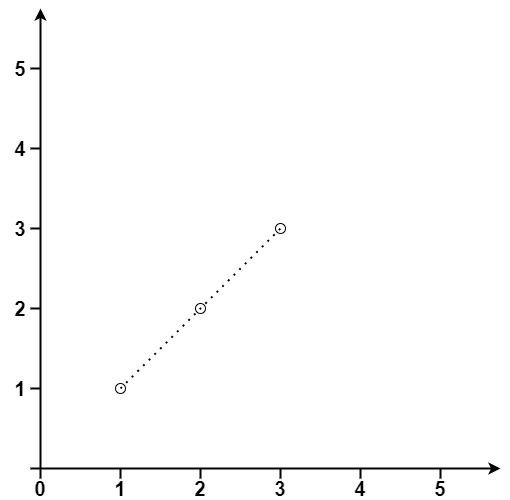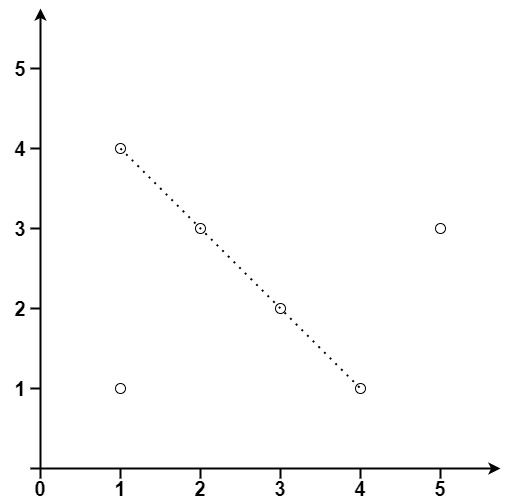# Question

Formatted question description: https://leetcode.ca/all/149.html

Given an array of points where points[i] = [xi, yi] represents a point on the X-Y plane, return the maximum number of points that lie on the same straight line.

Example 1:Input: points = [[1,1],[2,2],[3,3]]
Output: 3


Example 2:Input: points = [[1,1],[3,2],[5,3],[4,1],[2,3],[1,4]]
Output: 4


Constraints:

• 1 <= points.length <= 300
• points[i].length == 2
• -104 <= xi, yi <= 104
• All the points are unique.

# Algorithm

To detect collinear points in a given set of points, we can calculate the slope between every pair of points, as each slope represents a straight line. For each straight line, we can bring in all the points to see if they are collinear and calculate the number of collinear points.

Two special cases need to be considered. First, when two points coincide, a straight line cannot be determined, but this is also a collinear case and requires special treatment. Secondly, the case where the slope does not exist requires special treatment, as the slope k of the two points (x1, y1) and (x2, y2) is expressed as (y2-y1) / (x2-x1), and the slope does not exist when x1 = x2.

To keep track of the mapping between the slope and the number of collinear points, we can use a TreeMap. The first case of coincident points can assume its slope is INT_MIN, and the second case assumes its slope is INT_MAX, so TreeMap can be used for mapping. We also need to use a variable duplicate to record the number of coincident points. Finally, we just need to add the number of collinear points in the TreeMap to get the total number of collinear points.

Overall, we can use the following steps to detect collinear points:

1. Initialize a TreeMap to record the mapping between the slope and the number of collinear points.
2. Traverse every pair of points to calculate the slope.
3. If the slope does not exist, handle it as a special case and update the duplicate variable.
4. If the slope exists, update the number of collinear points in the TreeMap.
5. Add the duplicate variable to the number of collinear points in the TreeMap to get the total number of collinear points.

# Code

• import java.util.HashMap;
import java.util.Map;

public class Max_Points_on_a_Line {

public static void main(String[] args) {

double a = 0.0 / (-1);
System.out.println("a:" + a);

double b = 0.0 / (1);
System.out.println("b:" + b);

System.out.println("a == b ? " + (a == b)); // output: true

HashMap<Double, Integer> hm = new HashMap<Double, Integer>();
hm.put(a, 1);
hm.put(b, 1);
// @note:@memorize: so, different keys
System.out.println("hashmap keys: " + (hm.keySet())); // output: [-0.0, 0.0]

}

/**
* Definition for a point.
* class Point {
*     int x;
*     int y;
*     Point() { x = 0; y = 0; }
*     Point(int a, int b) { x = a; y = b; }
* }
*/
public class Solution {
public int maxPoints(Point[] points) {
if (points == null || points.length == 0)
return 0;

// line slope => number of points
HashMap<Double, Integer> hm = new HashMap<Double, Integer>();
int max = 0;

// have to touch every possible line, so O(logN)
for (int i = 0; i < points.length; i++) {
int duplicate = 1;
int vertical = 0;
for (int j = i + 1; j < points.length; j++) {
// @note:@memorize: handle duplicates and vertical
if (points[i].x == points[j].x) {
if (points[i].y == points[j].y) {
duplicate++; // itself
} else {
vertical++; // vertical line at the same x position
}
} else {
double slope = points[j].y == points[i].y ? 0.0 : (1.0 * (points[j].y - points[i].y)) / (points[j].x - points[i].x);

hm.merge(slope, 1, (a, b) -> a + b);
}
}

// record max number of points for one line
for (Integer count : hm.values()) {
if (count + duplicate > max) {
max = count + duplicate;
}
}

// @note:@memorize: special case for vertical line points
max = Math.max(vertical + duplicate, max);

hm.clear(); // @note: api, better than create a new hashmap?
}

return max;
}

}
}

############

class Solution {
public int maxPoints(int[][] points) {
int n = points.length;
int ans = 1;
for (int i = 0; i < n; ++i) {
int x1 = points[i], y1 = points[i];
Map<String, Integer> cnt = new HashMap<>();
for (int j = i + 1; j < n; ++j) {
int x2 = points[j], y2 = points[j];
int dx = x2 - x1, dy = y2 - y1;
int g = gcd(dx, dy);
String k = (dx / g) + "." + (dy / g);
cnt.put(k, cnt.getOrDefault(k, 0) + 1);
ans = Math.max(ans, cnt.get(k) + 1);
}
}
return ans;
}

private int gcd(int a, int b) {
return b == 0 ? a : gcd(b, a % b);
}
}

• // OJ: https://leetcode.com/problems/max-points-on-a-line/
// Time: O(N^3)
//   In worse case, when visiting ith point, there will be O(i^2)
//   lines, but all the lines are of constant size 2. So in
//   sum it's O(N^3), not O(N^4).
// Space: O(N^2)
namespace std {
template <> struct hash<Point> {
std::size_t operator () (const Point &p) const {
return hash<int>()(p.x) ^ hash<int>()(p.y);
}
};
}

bool operator==(const Point& a, const Point& b) {
return a.x == b.x && a.y == b.y;
}

class Solution {
private:
bool onSameLine(Point &a, Point &b, Point &c) {
return (long long)(a.x - b.x) * (a.y - c.y) == (long long)(a.x - c.x) * (a.y - b.y);
}
public:
int maxPoints(vector<Point>& points) {
if (points.size() <= 2) return points.size();
unordered_map<Point, int> m;
for (auto p : points) m[p]++;
vector<pair<Point, int>> ps(m.begin(), m.end());
vector<vector<int>> lines(1, vector<int>{ 0, 1 });
int N = ps.size();
for (int i = 2; i < N; ++i) {
auto &p = ps[i].first;
for (auto &line : lines) {
auto &p1 = ps[line].first, &p2 = ps[line].first;
if (!onSameLine(p1, p2, p)) continue;
for (int neighbor : line) bad[neighbor] = 0;
line.push_back(i);
}
for (int j = 0; j < i; ++j) {
if (bad[j]) lines.emplace_back(vector<int>{ j, i });
}
}
int ans = 2;
for (auto line : lines) {
int cnt = 0;
for (auto i : line) cnt += ps[i].second;
ans = max(ans, cnt);
}
return ans;
}
};

• class Solution:
def maxPoints(self, points: List[List[int]]) -> int:
def gcd(a, b):
return a if b == 0 else gcd(b, a % b)

n = len(points)
ans = 1
for i in range(n):
x1, y1 = points[i]
cnt = Counter()
for j in range(i + 1, n):
x2, y2 = points[j]
dx, dy = x2 - x1, y2 - y1
g = gcd(dx, dy)
k = (dx // g, dy // g)
cnt[k] += 1
ans = max(ans, cnt[k] + 1)
return ans

############

# Definition for a point.
# class Point(object):
#     def __init__(self, a=0, b=0):
#         self.x = a
#         self.y = b

class Solution(object):
def maxPoints(self, points):
"""
:type points: List[Point]
:rtype: int
"""

def gcd(a, b):
while b:
a, b = b, a % b
return a

ans = 1
d = {}
points.sort(key=lambda p: (p.x, p.y))
for i in range(0, len(points)):
if i > 0 and (points[i].x, points[i].y) == (points[i - 1].x, points[i - 1].y):
continue
overlap = 1
for j in range(i + 1, len(points)):
x1, y1 = points[i].x, points[i].y
x2, y2 = points[j].x, points[j].y
ku, kd = y2 - y1, x2 - x1
if (x1, y1) != (x2, y2):
kg = gcd(ku, kd)
ku /= kg
kd /= kg
d[(ku, kd, x1, y1)] = d.get((ku, kd, x1, y1), 0) + 1
else:
overlap += 1
ans = max(ans, overlap)
ans = max(ans, d.get((ku, kd, x1, y1), 0) + overlap)
return min(ans, len(points))


• func maxPoints(points [][]int) int {
n := len(points)
ans := 1
type pair struct{ x, y int }
for i := 0; i < n; i++ {
x1, y1 := points[i], points[i]
cnt := map[pair]int{}
for j := i + 1; j < n; j++ {
x2, y2 := points[j], points[j]
dx, dy := x2-x1, y2-y1
g := gcd(dx, dy)
k := pair{dx / g, dy / g}
cnt[k]++
if ans < cnt[k]+1 {
ans = cnt[k] + 1
}
}
}
return ans
}

func gcd(a, b int) int {
if b == 0 {
return a
}
return gcd(b, a%b)
}

• ﻿public class Solution {
public int MaxPoints(int[][] points) {
int n = points.Length;
int ans = 1;
for (int i = 0; i < n; ++i) {
int x1 = points[i], y1 = points[i];
for (int j = i + 1; j < n; ++j) {
int x2 = points[j], y2 = points[j];
int cnt = 2;
for (int k = j + 1; k < n; ++k) {
int x3 = points[k], y3 = points[k];
int a = (y2 - y1) * (x3 - x1);
int b = (y3 - y1) * (x2 - x1);
if (a == b) {
++cnt;
}
}
if (ans < cnt) {
ans = cnt;
}
}
}
return ans;
}
}